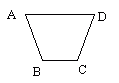Email us to get an instant 20% discount on highly effective K-12 Math & English kwizNET Programs!

#### Online Quiz (WorksheetABCD)

Questions Per Quiz = 2 4 6 8 10

### Grade 3 - Mathematics8.3 Polygons

 Polygons are closed figures that are formed by line segments. Each side is a line segment. The point where two line segments meet is a vertex. The sides do not cross each other and exactly two sides meet at every vertex. The segments that form the boundary are sides, which meet at a vertices to form the interior angle. If we connect two vertices that are not adjacent on the polygon, we form a diagonal. Examples: Squares, Triangles, Rectangles, etc. Directions: Answer the following questions. Also draw 10 examples of polygons an name the sides, vertices and diagonals on a sheet of paper.
 Q 1: Which of the following figures is not a polygon?DCAB Q 2: Polygons are named by starting at one vertex and naming all the other vertices in order. Name this figure.Polygon ACDBPolygon ABCDPolygon ADBC Q 3: In a polygon each side is aline segmentpointdot Q 4: Which of these is not a polygon?rectanglesquarecircletriangle Question 5: This question is available to subscribers only! Question 6: This question is available to subscribers only!